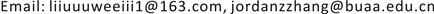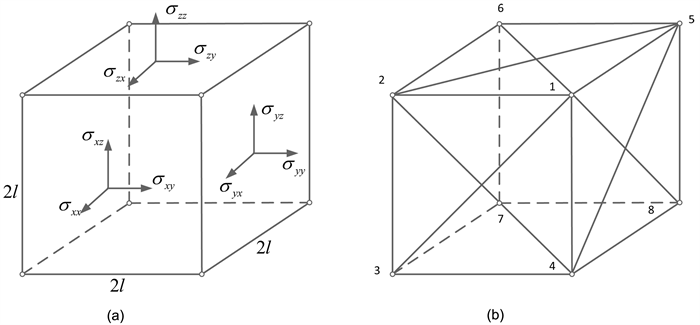﻿ 材料本构模拟的桁架模型和屈曲问题分析 Simulation of Material Constitution and Buckling Analysis Based on a Truss Model

International Journal of Mechanics Research
Vol.06 No.04(2017), Article ID:23044,12 pages
10.12677/IJM.2017.64019

Simulation of Material Constitution and Buckling Analysis Based on a Truss Model

Wei Liu, Zheng Zhang

Institute of Solid Mechanics, Beijing University of Aeronautics and Astronautics, BeijingReceived: Nov. 28th, 2017; accepted: Dec. 11th, 2017; published: Dec. 18th, 2017ABSTRACT

The mechanical behavior of the truss model is simulated, and the corresponding frame model is constructed for the orthogonal anisotropy and the isotropic material in this paper. The model parameters are determined according to the constitutive relation of the material (Hooke’s law). The influence of the Poisson’s ratio on the model structure validates the applicability of the model and the rationality of the truss model to simulate the mechanical behavior is also discussed. This paper uses the truss model to study the behavior of the material flexing for the isotropic material, the basic parameters of the truss model and the buckling behavior of the material bar are combined to establish the criterion of the flexion failure of the frame model. Failure of the material mesoscopic mechanism is finally discussed.

Keywords:Constitutive Relation, Truss Model, Poisson’s Ratio, Buckling, Mesomechanism1. 前言

2. 桁架模型

2.1. 桁架模型的构成

《断裂与损伤力学》(第二版)  采用如图1(b)所示桁架模型，模拟边长为2l的介质正方体元(参看图1(a))，设桁架模型边长也为2l，桁架包括棱边杆和面心对角杆两类杆件，其本构关系可表示为：

${N}_{eij}={K}_{eij}{\Delta }_{eij},\text{\hspace{0.17em}}\text{\hspace{0.17em}}\text{\hspace{0.17em}}{N}_{dij}={K}_{dij}{\Delta }_{dij}$ (2.1)Figure 1. The diagram of truss model. (a) Continuum unit; (b) Truss model

2.2. 桁架模型与介质单元的变形一致性

2.2.1. 桁架节点和介质单元的位移关系

$\text{d}{u}_{i}={\epsilon }_{ij}\text{d}{x}_{j}$ (2.2a)

${u}_{i}={\epsilon }_{ij}{x}_{j}$ (2.2b)

${u}_{i}^{1}=l\left(+{\epsilon }_{ix}+{\epsilon }_{iy}+{\epsilon }_{iz}\right),\text{\hspace{0.17em}}\text{\hspace{0.17em}}\text{\hspace{0.17em}}{u}_{i}^{2}=l\left(-{\epsilon }_{ix}+{\epsilon }_{iy}+{\epsilon }_{iz}\right),\text{\hspace{0.17em}}\text{\hspace{0.17em}}\text{\hspace{0.17em}}{u}_{i}^{3}=l\left(-{\epsilon }_{ix}-{\epsilon }_{iy}+{\epsilon }_{iz}\right)$

${u}_{i}^{4}=l\left(+{\epsilon }_{ix}-{\epsilon }_{iy}+{\epsilon }_{iz}\right),\text{\hspace{0.17em}}\text{\hspace{0.17em}}\text{\hspace{0.17em}}{u}_{i}^{5}=l\left(+{\epsilon }_{ix}+{\epsilon }_{iy}-{\epsilon }_{iz}\right),\text{\hspace{0.17em}}\text{\hspace{0.17em}}\text{\hspace{0.17em}}{u}_{i}^{6}=l\left(-{\epsilon }_{ix}+{\epsilon }_{iy}-{\epsilon }_{iz}\right)$

${u}_{i}^{7}=l\left(-{\epsilon }_{ix}-{\epsilon }_{iy}-{\epsilon }_{iz}\right),\text{\hspace{0.17em}}\text{\hspace{0.17em}}\text{\hspace{0.17em}}{u}_{i}^{8}=l\left(+{\epsilon }_{ix}-{\epsilon }_{iy}-{\epsilon }_{iz}\right)$ (2.3)

2.2.2. 桁架的虚功原理

$\delta {W}_{e}=\delta {W}_{i}$ (2.4)

$\delta {W}_{e}=8{l}^{3}\left({\sigma }_{xx}\delta {\epsilon }_{xx}+{\sigma }_{yy}\delta {\epsilon }_{yy}+{\sigma }_{zz}\delta {\epsilon }_{zz}\right)+16{l}^{3}\left({\sigma }_{xy}\delta {\epsilon }_{xy}+{\sigma }_{yz}\delta {\epsilon }_{yz}+{\sigma }_{zx}\delta {\epsilon }_{zx}\right)$ (2.5)

$\delta {W}_{i}=\delta {W}_{ie}+\delta {W}_{id}$ (2.6)

$\begin{array}{l}\delta {W}_{ie}={N}_{12}\delta {\Delta }_{12}+{N}_{34}\delta {\Delta }_{34}+{N}_{56}\delta {\Delta }_{56}+{N}_{78}\delta {\Delta }_{78}+{N}_{14}\delta {\Delta }_{14}+{N}_{23}\delta {\Delta }_{23}+{N}_{67}\delta {\Delta }_{67}\\ \text{\hspace{0.17em}}\text{\hspace{0.17em}}\text{\hspace{0.17em}}\text{\hspace{0.17em}}\text{\hspace{0.17em}}\text{\hspace{0.17em}}\text{\hspace{0.17em}}\text{\hspace{0.17em}}\text{\hspace{0.17em}}\text{\hspace{0.17em}}+{N}_{58}\delta {\Delta }_{58}+{N}_{15}\delta {\Delta }_{15}+{N}_{26}\delta {\Delta }_{26}+{N}_{37}\delta {\Delta }_{37}+{N}_{48}\delta {\Delta }_{48}\end{array}$

$\begin{array}{l}\delta {W}_{id}={N}_{13}\delta {\Delta }_{13}+{N}_{24}\delta {\Delta }_{24}+{N}_{57}\delta {\Delta }_{57}+{N}_{68}\delta {\Delta }_{68}+{N}_{27}\delta {\Delta }_{27}+{N}_{36}\delta {\Delta }_{36}+{N}_{38}\delta {\Delta }_{38}\\ \text{\hspace{0.17em}}\text{\hspace{0.17em}}\text{\hspace{0.17em}}\text{\hspace{0.17em}}\text{\hspace{0.17em}}\text{\hspace{0.17em}}\text{\hspace{0.17em}}\text{\hspace{0.17em}}\text{\hspace{0.17em}}\text{\hspace{0.17em}}+{N}_{47}\delta {\Delta }_{47}+{N}_{18}\delta {\Delta }_{18}+{N}_{45}\delta {\Delta }_{45}+{N}_{16}\delta {\Delta }_{16}+{N}_{25}\delta {\Delta }_{25}\end{array}$ (2.7)

2.2.3. 桁架的平衡方程(介质应力与桁架内力关系)

${\sigma }_{xx}=\frac{1}{{l}^{2}}\left[\frac{1}{4}\left({N}_{12}+{N}_{34}+{N}_{56}+{N}_{78}\right)+\frac{\sqrt{2}}{8}\left({N}_{13}+{N}_{24}+{N}_{16}+{N}_{25}+{N}_{57}+{N}_{68}+{N}_{47}+{N}_{38}\right)\right]$

${\sigma }_{yy}=\frac{1}{{l}^{2}}\left[\frac{1}{4}\left({N}_{14}+{N}_{23}+{N}_{58}+{N}_{67}\right)+\frac{\sqrt{2}}{8}\left({N}_{13}+{N}_{24}+{N}_{57}+{N}_{68}+{N}_{18}+{N}_{45}+{N}_{27}+{N}_{36}\right)\right]$

${\sigma }_{zz}=\frac{1}{{l}^{2}}\left[\frac{1}{4}\left({N}_{15}+{N}_{26}+{N}_{37}+{N}_{48}\right)+\frac{\sqrt{2}}{8}\left({N}_{16}+{N}_{25}+{N}_{47}+{N}_{38}+{N}_{18}+{N}_{45}+{N}_{27}+{N}_{36}\right)\right]$

${\sigma }_{yz}=\frac{1}{{l}^{2}}\frac{\sqrt{2}}{8}\left({N}_{18}-{N}_{45}+{N}_{27}-{N}_{36}\right)$

${\sigma }_{zx}=\frac{1}{{l}^{2}}\frac{\sqrt{2}}{8}\left({N}_{16}-{N}_{25}+{N}_{47}-{N}_{38}\right)$

${\sigma }_{xy}=\frac{1}{{l}^{2}}\frac{\sqrt{2}}{8}\left({N}_{13}-{N}_{24}+{N}_{57}-{N}_{68}\right)$ (2.8)

2.2.4. 桁架模型参数确定的一般方法

$\left(\begin{array}{c}{\sigma }_{xx}\\ {\sigma }_{yy}\\ {\sigma }_{zz}\\ {\sigma }_{yz}\\ {\sigma }_{xz}\\ {\sigma }_{xy}\end{array}\right)=\left(\begin{array}{cccccc}{C}_{11}& {C}_{12}& {C}_{13}& {C}_{14}& {C}_{15}& {C}_{16}\\ & {C}_{22}& {C}_{23}& {C}_{24}& {C}_{25}& {C}_{26}\\ & & {C}_{33}& {C}_{34}& {C}_{35}& {C}_{36}\\ & & & {C}_{44}& {C}_{45}& {C}_{46}\\ & \text{sys}& & & {C}_{55}& {C}_{56}\\ & & & & & {C}_{66}\end{array}\right)\left(\begin{array}{c}{\epsilon }_{xx}\\ {\epsilon }_{yy}\\ {\epsilon }_{zz}\\ {\epsilon }_{yz}\\ {\epsilon }_{xz}\\ {\epsilon }_{xy}\end{array}\right)$ (2.9)

$\begin{array}{c}{\sigma }_{xx}=\left(2{K}_{ex}+{K}_{dy}+{K}_{dz}\right){\epsilon }_{xx}+{K}_{dz}{\epsilon }_{yy}+{K}_{dy}{\epsilon }_{zz}+\frac{1}{4}\left({K}_{16}-{K}_{25}+{K}_{47}-{K}_{38}\right){\epsilon }_{zx}\\ \text{\hspace{0.17em}}\text{\hspace{0.17em}}+\frac{1}{4}\left({K}_{13}-{K}_{24}+{K}_{57}-{K}_{68}\right){\epsilon }_{xy}\end{array}$

$\begin{array}{c}{\sigma }_{yy}=\left(2{K}_{ey}+{K}_{dx}+{K}_{dz}\right){\epsilon }_{yy}+{K}_{dx}{\epsilon }_{zz}+{K}_{dz}{\epsilon }_{xx}+\frac{1}{4}\left({K}_{13}-{K}_{24}+{K}_{57}-{K}_{68}\right){\epsilon }_{xy}\\ \text{\hspace{0.17em}}\text{\hspace{0.17em}}+\frac{1}{4}\left({K}_{18}-{K}_{45}+{K}_{27}-{K}_{36}\right){\epsilon }_{yz}\end{array}$

$\begin{array}{c}{\sigma }_{zz}=\left(2{K}_{ez}+{K}_{dx}+{K}_{dx}\right){\epsilon }_{zz}+{K}_{dy}{\epsilon }_{xx}+{K}_{dx}{\epsilon }_{yy}+\frac{1}{4}\left({K}_{18}-{K}_{45}+{K}_{27}-{K}_{36}\right){\epsilon }_{yz}\\ \text{\hspace{0.17em}}\text{\hspace{0.17em}}+\frac{1}{4}\left({K}_{16}-{K}_{25}+{K}_{47}-{K}_{38}\right){\epsilon }_{zx}\end{array}$

${\sigma }_{zy}=\frac{1}{8}\left({K}_{18}-{K}_{45}+{K}_{27}-{K}_{36}\right)\left({\epsilon }_{yy}+{\epsilon }_{zz}\right)+{K}_{dx}{\epsilon }_{zy}$

${\sigma }_{xz}=\frac{1}{8}\left({K}_{16}-{K}_{25}+{K}_{47}-{K}_{38}\right)\left({\epsilon }_{xx}+{\epsilon }_{zz}\right)+{K}_{dy}{\epsilon }_{xz}$

${\sigma }_{xy}=\frac{1}{8}\left({K}_{13}-{K}_{24}+{K}_{57}-{K}_{68}\right)\left({\epsilon }_{xx}+{\epsilon }_{yy}\right)+{K}_{dz}{\epsilon }_{xy}$ (2.10)

${K}_{ex}=\frac{1}{4}\left({K}_{12}+{K}_{34}+{K}_{56}+{K}_{78}\right),\text{\hspace{0.17em}}\text{\hspace{0.17em}}\text{\hspace{0.17em}}{K}_{ey}=\frac{1}{4}\left({K}_{14}+{K}_{23}+{K}_{58}+{K}_{67}\right)$

${K}_{ez}=\frac{1}{4}\left({K}_{15}+{K}_{26}+{K}_{37}+{K}_{48}\right),\text{\hspace{0.17em}}\text{\hspace{0.17em}}\text{\hspace{0.17em}}{K}_{dx}=\frac{1}{4}\left({K}_{18}+{K}_{45}+{K}_{27}+{K}_{36}\right)$

${K}_{dy}=\frac{1}{4}\left({K}_{16}+{K}_{25}+{K}_{47}+{K}_{38}\right),\text{\hspace{0.17em}}\text{\hspace{0.17em}}\text{\hspace{0.17em}}{K}_{dz}=\frac{1}{4}\left({K}_{13}+{K}_{24}+{K}_{57}+{K}_{68}\right)$

3. 正交各向异性材料模拟–桁架模型参数确定

${\sigma }_{xx}={C}_{11}{\epsilon }_{xx}+{C}_{12}{\epsilon }_{yy}+{C}_{13}{\epsilon }_{zz}$

${\sigma }_{yy}={C}_{21}{\epsilon }_{xx}+{C}_{22}{\epsilon }_{yy}+{C}_{23}{\epsilon }_{zz}$

${\sigma }_{zz}={C}_{31}{\epsilon }_{xx}+{C}_{32}{\epsilon }_{yy}+{C}_{33}{\epsilon }_{zz}$

${\sigma }_{xy}={C}_{44}{\epsilon }_{xy}{\sigma }_{yz}={C}_{55}{\epsilon }_{yz}{\sigma }_{xz}={C}_{66}{\epsilon }_{xz}$ (3.1)

${C}_{11}=\frac{1-{\mu }_{23}{\mu }_{32}}{{E}_{1}{E}_{2}\Delta }{C}_{12}=\frac{{\mu }_{21}+{\mu }_{31}{\mu }_{23}}{{E}_{3}{E}_{2}\Delta }=\frac{{\mu }_{12}+{\mu }_{13}{\mu }_{32}}{{E}_{3}{E}_{2}\Delta }$

${C}_{22}=\frac{1-{\mu }_{13}{\mu }_{31}}{{E}_{1}{E}_{3}\Delta }{C}_{23}=\frac{{\mu }_{32}+{\mu }_{12}{\mu }_{31}}{{E}_{1}{E}_{3}\Delta }=\frac{{\mu }_{23}+{\mu }_{21}{\mu }_{13}}{{E}_{3}{E}_{1}\Delta }$

${C}_{33}=\frac{1-{\mu }_{12}{\mu }_{21}}{{E}_{1}{E}_{2}\Delta }{C}_{13}=\frac{{\mu }_{13}+{\mu }_{12}{\mu }_{23}}{{E}_{1}{E}_{2}\Delta }=\frac{{\mu }_{31}+{\mu }_{21}{\mu }_{32}}{{E}_{2}{E}_{1}\Delta }$

${C}_{44}={G}_{23}{C}_{55}={G}_{13}{C}_{66}={G}_{12}$

$\Delta =\frac{1}{{E}_{1}{E}_{2}{E}_{3}}|\begin{array}{ccc}1& -{\mu }_{21}& -{\mu }_{31}\\ -{\mu }_{12}& 1& -{\mu }_{32}\\ -{\mu }_{13}& -{\mu }_{23}& 1\end{array}|$ (3.2)

${K}_{13}-{K}_{24}+{K}_{57}-{K}_{68}=0$

${K}_{16}-{K}_{25}+{K}_{47}-{K}_{38}=0$

${K}_{18}-{K}_{45}+{K}_{27}-{K}_{36}=0$

${K}_{16}={K}_{25}={K}_{xz},\text{\hspace{0.17em}}\text{\hspace{0.17em}}\text{\hspace{0.17em}}{K}_{13}={K}_{24}={K}_{xy},\text{\hspace{0.17em}}\text{\hspace{0.17em}}\text{\hspace{0.17em}}{K}_{18}={K}_{45}={K}_{yz}$

${\sigma }_{xx}=\left[2{K}_{xx}+{K}_{xy}+{K}_{xz}\right]{\epsilon }_{xx}+{K}_{xy}{\epsilon }_{yy}+{K}_{xz}{\epsilon }_{zz}$

${\sigma }_{yy}={K}_{xy}{\epsilon }_{xx}+\left[2{K}_{yy}+{K}_{yz}+{K}_{yx}\right]{\epsilon }_{yy}+{K}_{yz}{\epsilon }_{zz}$

${\sigma }_{zz}={K}_{zx}{\epsilon }_{xx}+{K}_{zy}{\epsilon }_{yy}+\left[2{K}_{zz}+{K}_{yz}+{K}_{xz}\right]{\epsilon }_{zz}$

${\sigma }_{yz}=2{K}_{yz}{\epsilon }_{yz},\text{\hspace{0.17em}}\text{\hspace{0.17em}}\text{\hspace{0.17em}}{\sigma }_{xz}=2{K}_{xz}{\epsilon }_{xz},\text{\hspace{0.17em}}\text{\hspace{0.17em}}\text{\hspace{0.17em}}{\sigma }_{xy}=2{K}_{xy}{\epsilon }_{xy}$ (3.3)

$\text{}2{K}_{xx}+{K}_{xy}+{K}_{xz}={C}_{11}=\frac{1-{\mu }_{23}{\mu }_{32}}{{E}_{1}{E}_{2}\Delta }\text{,}\text{\hspace{0.17em}}\text{\hspace{0.17em}}{K}_{xy}={C}_{12}=\frac{{\mu }_{21}+{\mu }_{31}{\mu }_{23}}{{E}_{3}{E}_{2}\Delta }=\frac{{\mu }_{12}+{\mu }_{13}{\mu }_{32}}{{E}_{3}{E}_{2}\Delta }$

$2{K}_{yy}+{K}_{yz}+{K}_{yx}={C}_{22}=\frac{1-{\mu }_{13}{\mu }_{31}}{{E}_{1}{E}_{3}\Delta }\text{,}\text{\hspace{0.17em}}\text{\hspace{0.17em}}{K}_{zy}={C}_{23}=\frac{{\mu }_{32}+{\mu }_{12}{\mu }_{31}}{{E}_{1}{E}_{3}\Delta }=\frac{{\mu }_{23}+{\mu }_{21}{\mu }_{13}}{{E}_{3}{E}_{1}\Delta }$

$2{K}_{zz}+{K}_{yz}+{K}_{xz}={C}_{33}=\frac{1-{\mu }_{12}{\mu }_{21}}{{E}_{1}{E}_{2}\Delta }\text{,}\text{\hspace{0.17em}}\text{\hspace{0.17em}}{K}_{zx}={C}_{13}=\frac{{\mu }_{13}+{\mu }_{12}{\mu }_{23}}{{E}_{1}{E}_{2}\Delta }=\frac{{\mu }_{31}+{\mu }_{21}{\mu }_{32}}{{E}_{2}{E}_{1}\Delta }$ (3.4)

4. 各向同性材料模拟―改进的桁架模型

${E}_{i}=E,\text{\hspace{0.17em}}\text{\hspace{0.17em}}{\mu }_{ij}=\mu ,\text{\hspace{0.17em}}\text{\hspace{0.17em}}\text{\hspace{0.17em}}i,j=1,2,3$

${K}_{xy}={K}_{zy}={K}_{zx}=\frac{\mu }{\left(1-2\mu \right)\left(1+\mu \right)}E=\frac{1}{2\left(1+\mu \right)}E$ (4.1)

${\sigma }_{xx}=\left(2{K}_{e}+{K}_{d}\right){\epsilon }_{xx}+{K}_{d}\theta ,\text{\hspace{0.17em}}\text{\hspace{0.17em}}{\sigma }_{yy}=\left(2{K}_{e}+{K}_{d}\right){\epsilon }_{yy}+{K}_{d}\theta ,\text{\hspace{0.17em}}\text{\hspace{0.17em}}{\sigma }_{zz}=\left(2{K}_{e}+{K}_{d}\right){\epsilon }_{zz}+{K}_{d}\theta$

${\sigma }_{xy}=\left(2{K}_{d}+\frac{t}{l}{G}_{m}\right){\epsilon }_{xy},\text{\hspace{0.17em}}\text{\hspace{0.17em}}{\sigma }_{xz}=\left(2{K}_{d}+\frac{t}{l}{G}_{m}\right){\epsilon }_{xz},\text{\hspace{0.17em}}\text{\hspace{0.17em}}{\sigma }_{zy}=\left(2{K}_{d}+\frac{t}{l}{G}_{m}\right){\epsilon }_{zy}$ (4.2)

$\theta ={\epsilon }_{xx}+{\epsilon }_{yy}+{\epsilon }_{zz}$

${\sigma }_{xx}=\frac{E}{1+v}{\epsilon }_{xx}+\frac{Ev}{\left(1+v\right)\left(1-2v\right)}\theta$

${\sigma }_{yy}=\frac{E}{1+v}{\epsilon }_{yy}+\frac{Ev}{\left(1+v\right)\left(1-2v\right)}\theta$

${\sigma }_{zz}=\frac{E}{1+v}{\epsilon }_{zz}+\frac{Ev}{\left(1+v\right)\left(1-2v\right)}\theta$

${\sigma }_{xy}=\frac{E}{2\left(1+v\right)}{\epsilon }_{xy}$

${\sigma }_{xz}=\frac{E}{2\left(1+v\right)}{\epsilon }_{xz}$

${\sigma }_{yz}=\frac{E}{2\left(1+v\right)}{\epsilon }_{yz}$

$2{K}_{e}+{K}_{d}=\frac{E}{1+v},\text{\hspace{0.17em}}\text{\hspace{0.17em}}{K}_{d}=\frac{Ev}{\left(1+v\right)\left(1-2v\right)},\text{\hspace{0.17em}}\text{\hspace{0.17em}}2{K}_{d}+\frac{t}{l}{G}_{m}=\frac{E}{2\left(1+v\right)}$ (4.3)

${K}_{e}={K}_{xx}={K}_{yy}={K}_{zz}=\frac{1-3\mu }{2\left(1-2\mu \right)\left(1+\mu \right)}E$

${K}_{d}={K}_{xy}={K}_{zy}={K}_{zx}=\frac{\mu }{\left(1-2\mu \right)\left(1+\mu \right)}E$

${G}_{m}=\frac{l\left(1-4\mu \right)}{t\left(1-2\mu \right)\left(1+\mu \right)}E$ (4.4)

5. 屈曲问题中框架模型的受力形式

5.1. 杆的应力与微元体应力关系

${E}_{e}=\frac{\frac{1-3\mu }{\left(1-2\mu \right)\left(1+\mu \right)}E{l}^{2}}{{A}_{e}}={E}_{d}=\frac{\frac{\mu }{\left(1-2\mu \right)\left(1+\mu \right)}\sqrt{2}E{l}^{2}}{{A}_{d}}=\frac{E{l}^{2}}{A\left(1-2\mu \right)\left(1+\mu \right)}$ (5.1)

${\sigma }_{xx}=\frac{1}{{l}^{2}}\left[\frac{1}{4}\left({N}_{12}+{N}_{34}+{N}_{56}+{N}_{78}\right)+\frac{\sqrt{2}}{8}\left({N}_{13}+{N}_{24}+{N}_{16}+{N}_{25}+{N}_{57}+{N}_{68}+{N}_{47}+{N}_{38}\right)\right]$

${\sigma }_{yy}=\frac{1}{{l}^{2}}\left[\frac{1}{4}\left({N}_{14}+{N}_{23}+{N}_{58}+{N}_{67}\right)+\frac{\sqrt{2}}{8}\left({N}_{13}+{N}_{24}+{N}_{57}+{N}_{68}+{N}_{18}+{N}_{45}+{N}_{27}+{N}_{36}\right)\right]$

${\sigma }_{zz}=\frac{1}{{l}^{2}}\left[\frac{1}{4}\left({N}_{15}+{N}_{26}+{N}_{37}+{N}_{48}\right)+\frac{\sqrt{2}}{8}\left({N}_{16}+{N}_{25}+{N}_{47}+{N}_{38}+{N}_{18}+{N}_{45}+{N}_{27}+{N}_{36}\right)\right]$

${\sigma }_{yz}=\frac{1}{{l}^{2}}\frac{\sqrt{2}}{8}\left({N}_{18}-{N}_{45}+{N}_{27}-{N}_{36}\right)$

${\sigma }_{zx}=\frac{1}{{l}^{2}}\frac{\sqrt{2}}{8}\left({N}_{16}-{N}_{25}+{N}_{47}-{N}_{38}\right)$

${\sigma }_{xy}=\frac{1}{{l}^{2}}\frac{\sqrt{2}}{8}\left({N}_{13}-{N}_{24}+{N}_{57}-{N}_{68}\right)$ (5.2)

${\sigma }_{exx}=\frac{{l}^{2}}{A}\frac{1}{\left(1-2\mu \right)\left(1+\mu \right)}\left[{\sigma }_{xx}-\mu \left({\sigma }_{yy}+{\sigma }_{zz}\right)\right]$

${\sigma }_{eyy}=\frac{{l}^{2}}{A}\frac{1}{\left(1-2\mu \right)\left(1+\mu \right)}\left[{\sigma }_{yy}-\mu \left({\sigma }_{xx}+{\sigma }_{zz}\right)\right]$

${\sigma }_{ezz}=\frac{{l}^{2}}{A}\frac{1}{\left(1-2\mu \right)\left(1+\mu \right)}\left[{\sigma }_{zz}-\mu \left({\sigma }_{yy}+{\sigma }_{xx}\right)\right]$

${\sigma }_{13}={\sigma }_{57}=\frac{{l}^{2}}{A}\frac{1}{\left(1-2\mu \right)\left(1+\mu \right)}\left[\left(1-\mu \right)\left({\sigma }_{xx}+{\sigma }_{yy}\right)-2\mu {\sigma }_{zz}+2\left(1+\mu \right){\sigma }_{xy}\right]$

${\sigma }_{24}={\sigma }_{68}=\frac{{l}^{2}}{A}\frac{1}{\left(1-2\mu \right)\left(1+\mu \right)}\left[\left(1-\mu \right)\left({\sigma }_{xx}+{\sigma }_{yy}\right)-2\mu {\sigma }_{zz}-2\left(1+\mu \right){\sigma }_{xy}\right]$

${\sigma }_{18}={\sigma }_{27}=\frac{{l}^{2}}{A}\frac{1}{\left(1-2\mu \right)\left(1+\mu \right)}\left[\left(1-\mu \right)\left({\sigma }_{zz}+{\sigma }_{yy}\right)-2\mu {\sigma }_{xx}+2\left(1+\mu \right){\sigma }_{yz}\right]$

${\sigma }_{45}={\sigma }_{36}=\frac{{l}^{2}}{A}\frac{1}{\left(1-2\mu \right)\left(1+\mu \right)}\left[\left(1-\mu \right)\left({\sigma }_{zz}+{\sigma }_{yy}\right)-2\mu {\sigma }_{xx}-2\left(1+\mu \right){\sigma }_{yz}\right]$

${\sigma }_{16}={\sigma }_{47}=\frac{{l}^{2}}{A}\frac{1}{\left(1-2\mu \right)\left(1+\mu \right)}\left[\left(1-\mu \right)\left({\sigma }_{zz}+{\sigma }_{xx}\right)-2\mu {\sigma }_{yy}+2\left(1+\mu \right){\sigma }_{xz}\right]$

${\sigma }_{25}={\sigma }_{38}=\frac{{l}^{2}}{A}\frac{1}{\left(1-2\mu \right)\left(1+\mu \right)}\left[\left(1-\mu \right)\left({\sigma }_{zz}+{\sigma }_{xx}\right)-2\mu {\sigma }_{yy}-2\left(1+\mu \right){\sigma }_{xz}\right]$ (5.3)

5.2. 宏观单向压应力情况下框架模型受力形式

${\sigma }_{exx}=\frac{{l}^{2}}{A}\frac{1}{\left(1-2\mu \right)\left(1+\mu \right)}{\sigma }_{xx}$

${\sigma }_{eyy}=\frac{{l}^{2}}{A}\frac{1}{\left(1-2\mu \right)\left(1+\mu \right)}\left(-\mu {\sigma }_{xx}\right)$

${\sigma }_{ezz}=\frac{{l}^{2}}{A}\frac{1}{\left(1-2\mu \right)\left(1+\mu \right)}\left(-\mu {\sigma }_{xx}\right)$

${\sigma }_{13}={\sigma }_{57}=\frac{{l}^{2}}{A}\frac{1}{\left(1-2\mu \right)\left(1+\mu \right)}\left(1-\mu \right){\sigma }_{xx}$

${\sigma }_{24}={\sigma }_{68}=\frac{{l}^{2}}{A}\frac{1}{\left(1-2\mu \right)\left(1+\mu \right)}\left(1-\mu \right){\sigma }_{xx}$

${\sigma }_{18}={\sigma }_{27}=\frac{{l}^{2}}{A}\frac{1}{\left(1-2\mu \right)\left(1+\mu \right)}\left(-2\mu {\sigma }_{xx}\right)$

${\sigma }_{45}={\sigma }_{36}=\frac{{l}^{2}}{A}\frac{1}{\left(1-2\mu \right)\left(1+\mu \right)}\left(-2\mu {\sigma }_{xx}\right)$

${\sigma }_{16}={\sigma }_{47}=\frac{{l}^{2}}{A}\frac{1}{\left(1-2\mu \right)\left(1+\mu \right)}\left(1-\mu \right){\sigma }_{xx}$

${\sigma }_{25}={\sigma }_{38}=\frac{{l}^{2}}{A}\frac{1}{\left(1-2\mu \right)\left(1+\mu \right)}\left(1-\mu \right){\sigma }_{xx}$ (5.4)

1) 当某点处于单向应力状态时，各杆的受力状态与被模拟材料的参数有关，也与构件的具体尺寸有关。公式中的 ${l}^{2}/A$ 项中 代表了框架模型边长的1/2，故各个杆件的应力数值受杆件的尺寸比例取值影响。

2) 由于被模拟材料的宏观失效形式是杆件屈曲，故在微观上我们亦考察与宏观失效形式相一致的框架模型杆件受压失效形式，而不考虑杆件受拉应力的失效形式。根据式(5.1)，棱边杆的弹性模量为

${E}_{e}=\frac{\frac{1-3\mu }{\left(1-2\mu \right)\left(1+\mu \right)}E{l}^{2}}{{A}_{e}}$ (5.5)

a) 在 $0<\mu <1/3$ 时，棱边杆的弹性模量为正数，与宏观杆屈曲的受力失效形式一致；

b) 在 $1/3<\mu <1/2$ 时，棱边杆的弹性模量为负数，与客观材料情况不一致，不做失效考虑。

3) 应该明确的是，由于构件的材料参数相同，故判别构件失效的依据为压应力较大的构件首先失效，进而建立框架模型的失效判据。故

a) 当 $0<\mu <1/3$ 时，最大的受力杆是与受力方向平行的棱边杆，应力为 ${\sigma }_{exx}$ ，即被模拟材料发生屈曲时，首先发生失效的是的棱边杆；

b) 当 $1/3<\mu <1/2$ 时，与受力方向相平行的面中的面内杆压应力最大，应力为

$\frac{{l}^{2}}{A}\frac{1}{\left(1-2\mu \right)\left(1+\mu \right)}\left(1-\mu \right){\sigma }_{xx}$

5.3. 宏观三向压应力情况下框架模型受力形式

${\sigma }_{xx}={\sigma }_{yy}={\sigma }_{zz}\left(\ne 0\right),\text{\hspace{0.17em}}\text{\hspace{0.17em}}{\sigma }_{yz}={\sigma }_{xy}={\sigma }_{xz}=0$

$\frac{{l}^{2}}{A}\frac{1}{\left(1-2\mu \right)\left(1+\mu \right)}\left(1-\mu \right){\sigma }_{xx}$

${\sigma }_{13}={\sigma }_{57}={\sigma }_{24}={\sigma }_{68}={\sigma }_{18}={\sigma }_{27}={\sigma }_{45}={\sigma }_{36}={\sigma }_{16}={\sigma }_{47}={\sigma }_{25}={\sigma }_{38}=\frac{{l}^{2}}{A}\frac{2}{1+\mu }{\sigma }_{xx}$ (5.6)

5.4. 宏观杆件屈曲时截面受力形式

1) 若 $0<\mu <1/3$ ，首先发生失效的是的棱边杆，根据式(5.4)，其应力为 $\frac{{l}^{2}}{A}\frac{1}{\left(1-2\mu \right)\left(1+\mu \right)}{\sigma }_{xx}$

2) 若 $1/3<\mu <1/2$ ，首先发生失效的是与受力方向相平行的面中的面内杆，根据式(5.4)，应力为

$\frac{{l}^{2}}{A}\frac{1}{\left(1-2\mu \right)\left(1+\mu \right)}\left(1-\mu \right){\sigma }_{xx}$

1) $0<\mu <1/4$ 时，在宏观材料杆件横截面中心部分所对应的框架模型中，与受力方向相平行的面中的面内杆应力最大首先失效，即宏观材料杆件横截面中心部分先于边缘部分失效。

2) $1/4<\mu <1/3$ 时，在宏观材料杆件横截面边缘部分所对应的框架模型中，与受力方向相平行棱边杆应力最大首先失效，即宏观材料杆件横截面边缘部分先于中心部分失效。

3) $1/3<\mu <1/2$ 时，在宏观材料杆件横截面边缘部分所对应的框架模型中，与受力方向相平行的面中的面内杆应力最大首先失效，即宏观材料杆件横截面边缘部分先于中心部分失效。

5.5. 本章小结

6. 本文小结

1) 对于各向同性材料，本文在原模型的基础上添加了只承受剪切的面板元件，解决了桁架模型对材料泊松效应的模拟问题。

2) 由改进的桁架模拟模型可知，引入的剪切板材料剪切板模量 ${G}_{m}=0$ 时，被模拟的材料泊松比为0.25；值得注意到的是，当材料的泊松比大于0.25且小于0.5时，桁架模型剪切板的材料剪切模量为负，即该剪切板的应力应变方向相反。

3) 基于框架模型的建立继而分析屈曲变化的引发机制，并结合框架模型计算对屈曲问题进行分析，讨论细长构件在压缩载荷下从压缩变形到弯曲变形的变化过程和力学作用，特别是在临界屈曲载荷下，分析框架模型的受力状况。

4) 从模型失效研究中我们看到，对于宏观杆材料的不同泊松比，框架模型的失效形式是不同的，由此可以反映出该宏观杆材料屈曲特性的不同。我们认为框架模型棱边杆失效时，宏观杆屈曲是由压应力主导的；框架模型与受力方向相平行的面中的面内杆失效时，宏观杆屈曲是由相应的剪切应力主导的。

Simulation of Material Constitution and Buckling Analysis Based on a Truss Model[J]. 力学研究, 2017, 06(04): 180-191. http://dx.doi.org/10.12677/IJM.2017.64019

1. 1. Schmit, L.A. (1960) Structural Design by Systematic Synthesis. 2nd Conference on Electronic Computation, ASCE, New York, 105-122.

2. 2. Cornell, C.A. (1968) Examples of Optimization Design: An Introduction to Structural Optimization. Solid Mechanics Division, University of Waterloo, Waterloo.

3. 3. Bendsoe, M.P., Bed-Tal, A. and Zowe, J. (1994) Optimization Methods for Truss Geometry and Topology Design. Structural Optimization, 17, 981-1002. https://doi.org/10.1007/BF01742459

4. 4. 刘亮, 翟宇毅. 桁架结构重分析的一种新方法[J]. 计算力学学报, 1998, 5(2): 149-152.

5. 5. Cheng, G.D. and Xu, G. (1997) ε-Relaxed Approach Structural Topology Optimization. Structural Optimization, 13, 152-166. https://doi.org/10.1007/BF01197454

6. 6. 张行. 断裂与损伤力学[M]. 第二版. 北京: 北京航空航天大学出版社, 2009.

7. 7. 王耀先. 复合材料力学与结构设计[M]. 上海: 华东理工大学出版社, 2012.